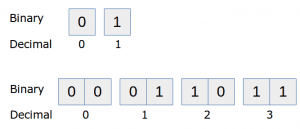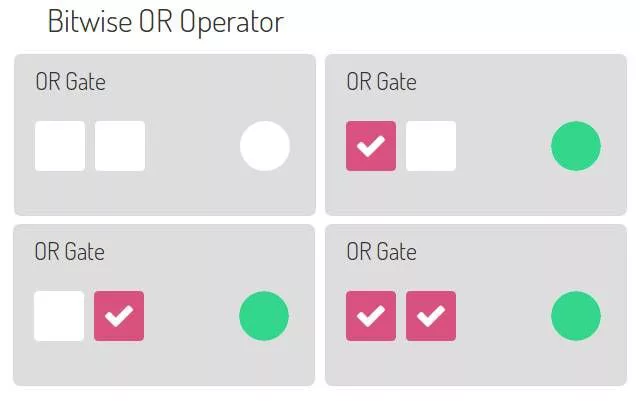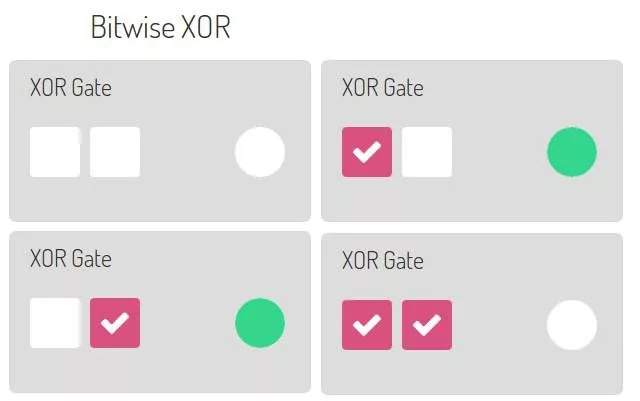# Python 二进制数和逻辑运算符

## 二进制数print int('01', 2)
print int('10', 2)
print int('11', 2)


print int('00000011', 2)   # outputs 3
print int('00010001', 2)   # outputs 17
print int('11111111', 2)   # outputs 255


## 二进制数的逻辑运算

0 1 0 1
1 0 1 0

inputA = int('0101',2)

print "Before shifting " + str(inputA) + " " + bin(inputA)
print "After shifting in binary: " + bin(inputA << 1)
print "After shifting in decimal: " + str(inputA << 1)


Before shifting 5 0b101
After shifting in binary: 0b1010
After shifting in decimal: 10


## Python 逻辑与运算符inputB = 1
print inputA & inputB   # Bitwise AND


inputA = int('00100011',2)   # define binary sequence inputA
inputB = int('00101101',2)   # define binary sequence inputB

print bin(inputA & inputB)   # logical AND on inputA and inputB and output in binary


0b100001   # equals 00100001


00100011
00101101
--------   Logical bitwise AND
00100001


## Python 逻辑或运算符inputA = int('00100011',2)  # define binary number
inputB = int('00101101',2)  # define binary number

print bin(inputA)            # prints inputA in binary
print bin(inputB)            # prints inputB in binary
print bin(inputA | inputB)   # Execute bitwise logical OR and print result in binary


0b100011
0b101101
0b101111


## XOR 运算符inputA = int('00100011',2)  # define binary number
inputB = int('00101101',2)  # define binary number

print bin(inputA)            # prints inputA in binary
print bin(inputB)            # prints inputB in binary
print bin(inputA ^ inputB)   # Execute bitwise logical OR and print result in binary


0b100011
0b101101
0b1110